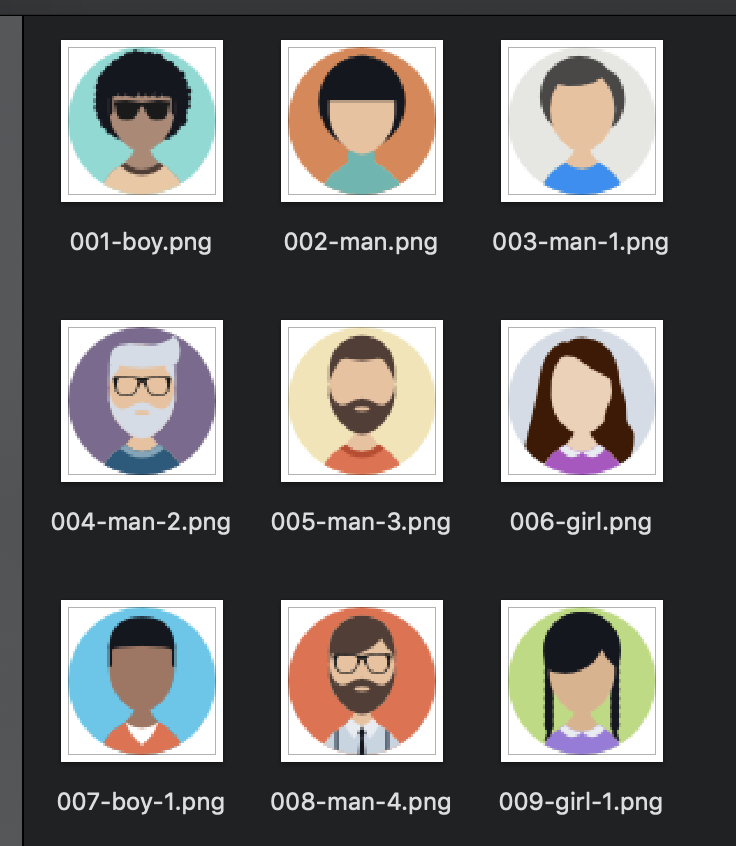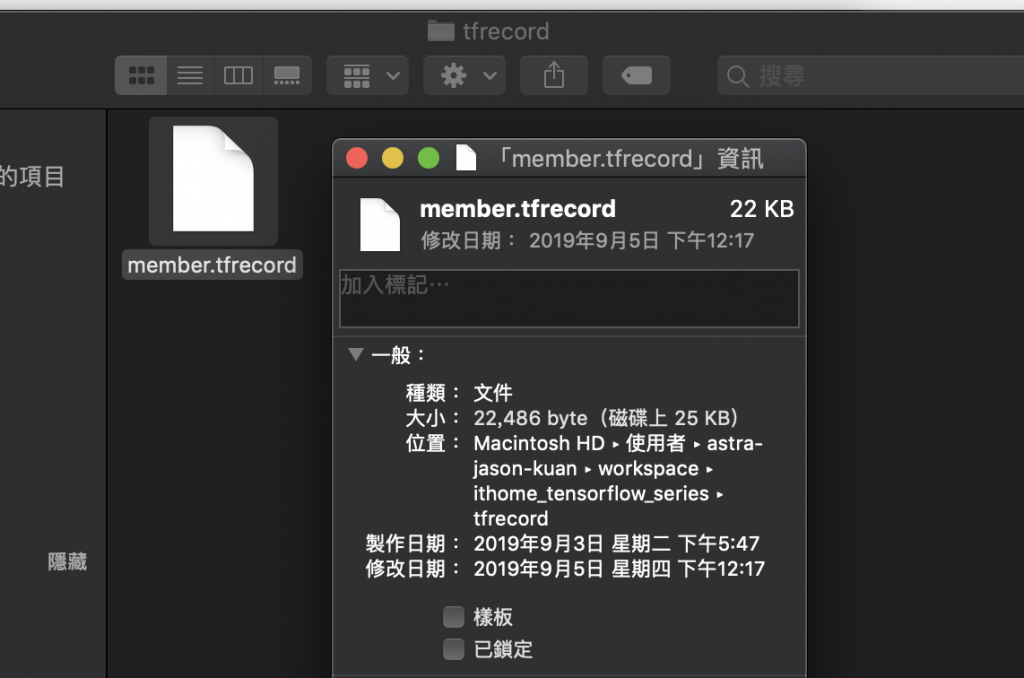DAY 12
0
Google Developers Machine Learning

【12】tensorflow 資料集應用：製作 tfrecord 篇

name_list = ['A', 'B', 'C', 'D', 'E', 'F', 'G', 'H', 'I']
image_list = ['001-boy.png', '002-man.png', '003-man-1.png',
'004-man-2.png', '005-man-3.png', '006-girl.png',
'007-boy-1.png', '008-man-4.png', '009-girl-1.png']
age_list = [12, 33, 25, 55, 40, 31, 14, 37, 10]
height_list = [140.2, 174.6, 165.1, 170.9, 168.2, 177.8, 153.1, 164.3, 134.1]
prefer_prods_list = [[1, 2], [1, 5], , [3, 4], [1, 3, 5], , [], [1, 2], [2, 4]]writer = tf.python_io.TFRecordWriter('../tfrecord/member.tfrecord')

for i, name in enumerate(name_list):
member_name = name.encode('utf8')
image = image_list[i]
age = age_list[i]
height = height_list[i]
prefer_prods = prefer_prods_list[i]

with tf.gfile.GFile(os.path.join('my-icons-collection', 'png', image), 'rb') as fid:

def int64_feature(value):
return tf.train.Feature(int64_list=tf.train.Int64List(value=[value]))

def int64_list_feature(value):
return tf.train.Feature(int64_list=tf.train.Int64List(value=value))

def bytes_feature(value):
return tf.train.Feature(bytes_list=tf.train.BytesList(value=[value]))

def float_feature(value):
return tf.train.Feature(float_list=tf.train.FloatList(value=[value]))

def data_to_example(name, encoded_image, age, height, prefer_prods):
example = tf.train.Example(features=tf.train.Features(feature={
'member/name': bytes_feature(name),
'member/encoded': bytes_feature(encoded_image),
'member/age': int64_feature(age),
'member/height': float_feature(height),
'member/prefer_prods': int64_list_feature(prefer_prods),
}))
return example

tf_example = data_to_example(member_name, encoded_image, age, height, prefer_prods)
writer.write(tf_example.SerializeToString())github原始碼